Maxim MAX4014 MAX4250

Accurate op amps have high open-loop gain, low offset voltage and current, low voltage and current noise, and low distortion. However, they often lack the ability to provide high output currents while maintaining all the other high-accuracy specifications. In other words, high-accuracy op amps have a problem driving low-impedance loads. One solution to the problem is to ’cancel' the load. If your resistive load is R Ω and you connect it in parallel with a negative resistor of –R Ω, the resistance of the parallel combination is infinite. The circuit of Figure 1 can generate negative resistance at its input: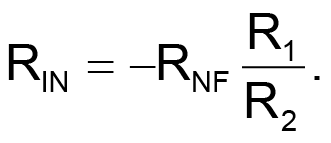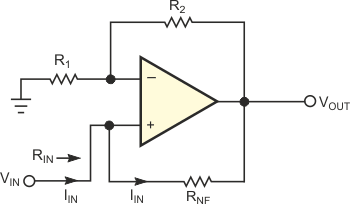Figure 1. This circuit exhibits negative resistance at its input.

You derive this value as follows: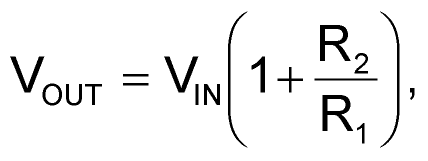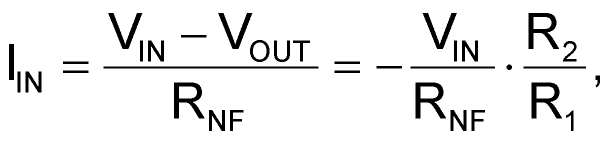and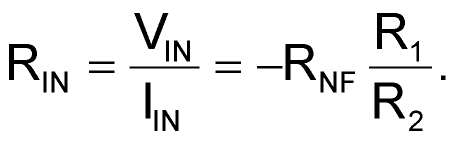Figure 2 presents a practical application of the concept. The first op amp is an accurate, unity-gain buffer, and the second op amp is a high-current, high-bandwidth, gain-of-2 driver. Because R1 = R2 in this negative-resistance stage, its input resistance is –RNF = –200 Ω, which matches the magnitude of the accurate buffer's load resistance. If these magnitudes match perfectly, the buffer sees an open circuit at its output. The buffer drives the positive input of the second amplifier, and the second amplifier, via its negative-resistance input, drives the load. Gain error, output-current limits, and resistor mismatches limit the minimum resistance the circuit can drive, but driving a 200 Ω load is easy. That load is an order of magnitude lower than the load the unassisted accurate amplifier can handle without suffering degradation of performance. Note that the second op amp's gain error, offset voltage, and offset current do not affect the first op amp's accuracy. The step response of this circuit is well-behaved and exhibits no ringing.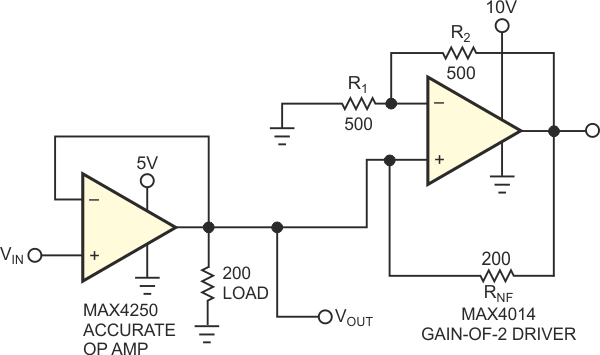Figure 2. Connecting a negative resistance in parallel with the load enables a precision op amp to drive 200 V.

The negative-resistance approach works equally well with dual-supply op amps, because the negative-resistance portion can both source and sink current. If the driver op amp does not have built-in gain-setting resistors, you can set its noninverting gain closer to unity, thereby allowing both op amps to share a power supply. This approach limits the output swing of the accurate op amp, but that restriction may be acceptable in a given application. To ensure full bandwidth for the accurate op amp, the driver op amp should have much higher bandwidth.

Materials on the topic

EDN